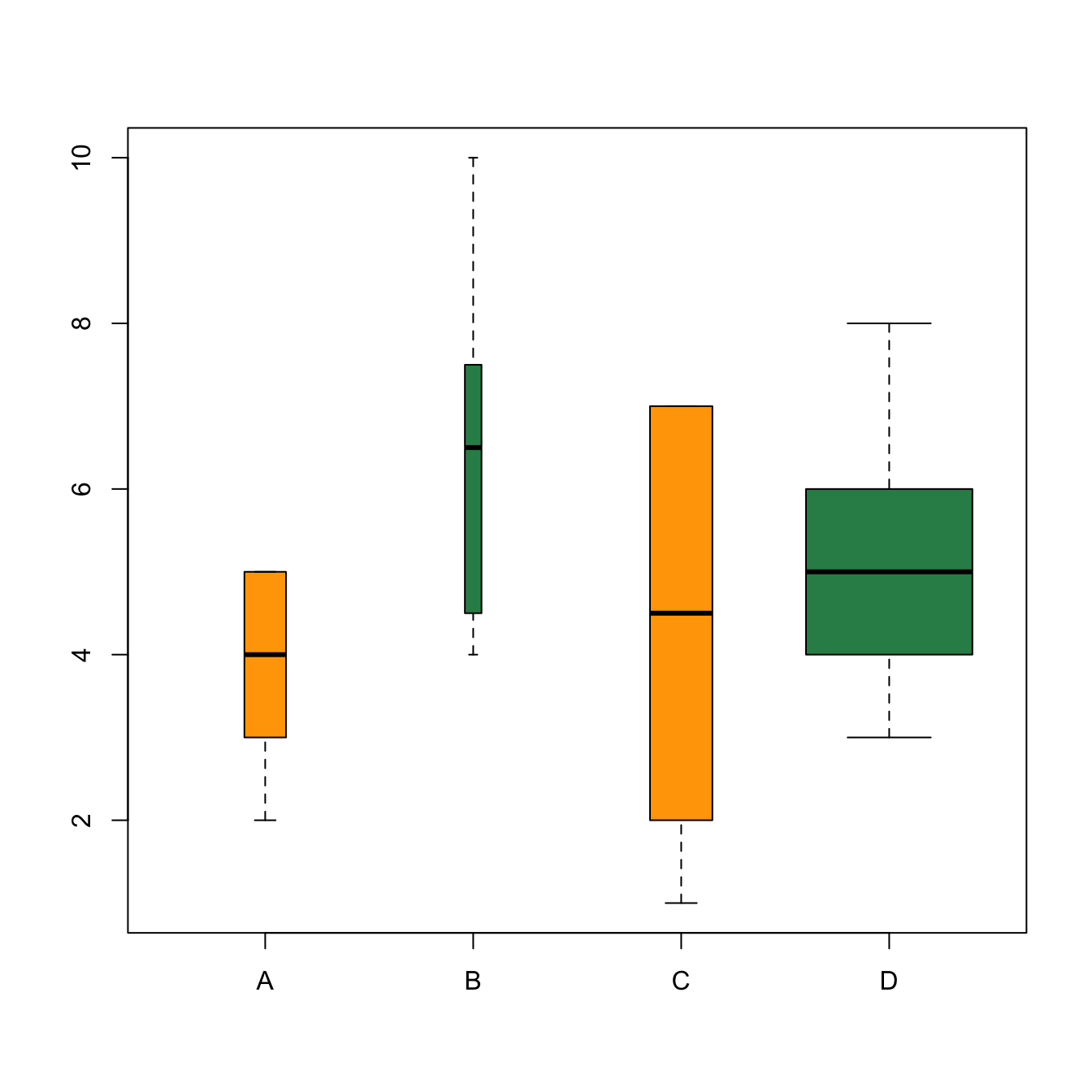# Boxplot with variable width

This examples demonstrates how to build a boxplot with variable width. It is useful to indicate what sample size is hidden behind each box. It is a base R implementation, see here for a ggplot2 version.

When the sample size behind each category is highly variable, it can be great to represent it through the box widths.

First calculate the proportion of each level using the `table()` function. Using these proportions will make the box twice bigger if a level is twice more represented. Then give these proportions to the `width` argument when you call the `boxplot()` function.``````# Dummy data
names <- c(rep("A", 20) , rep("B", 8) , rep("C", 30), rep("D", 80))
value <- c( sample(2:5, 20 , replace=T) , sample(4:10, 8 , replace=T),
sample(1:7, 30 , replace=T), sample(3:8, 80 , replace=T) )
data <- data.frame(names,value)

# Calculate proportion of each level
proportion <- table(data\$names)/nrow(data)

#Draw the boxplot, with the width proportionnal to the occurence !
boxplot(data\$value ~ data\$names , width=proportion , col=c("orange" , "seagreen"))``````

Related chart types

## Contact

This document is a work by Yan Holtz. Any feedback is highly encouraged. You can fill an issue on Github, drop me a message on Twitter, or send an email pasting yan.holtz.data with gmail.com.